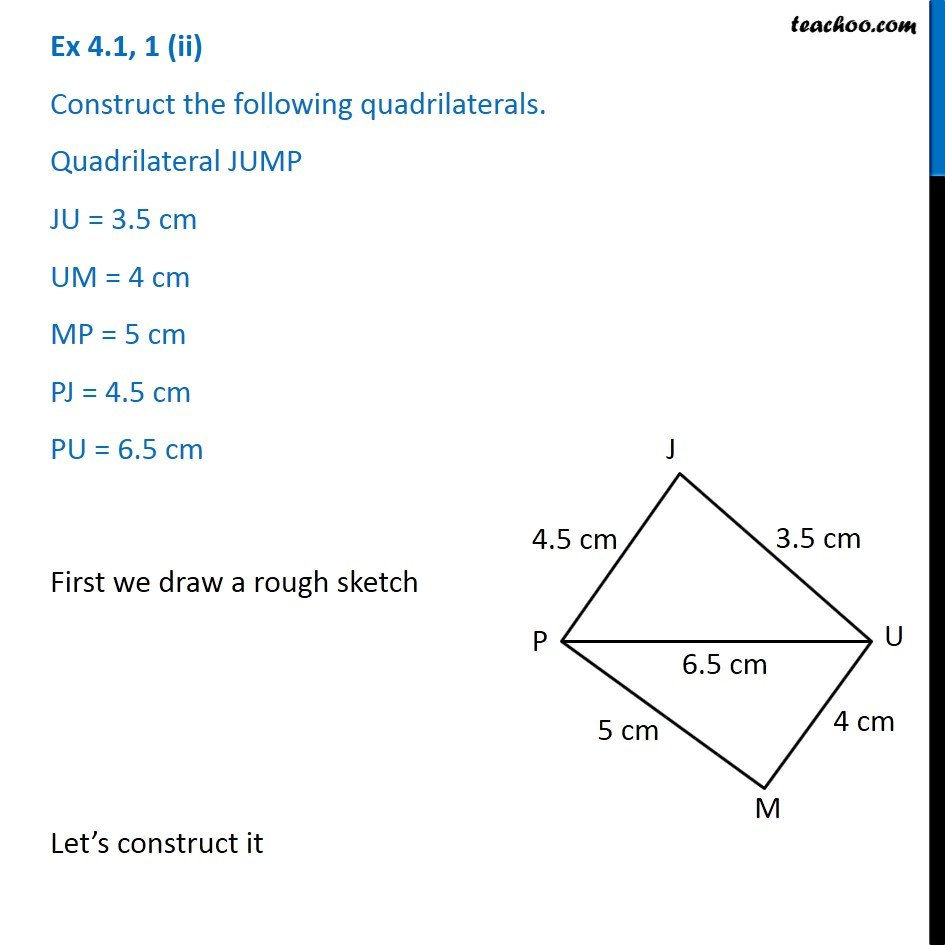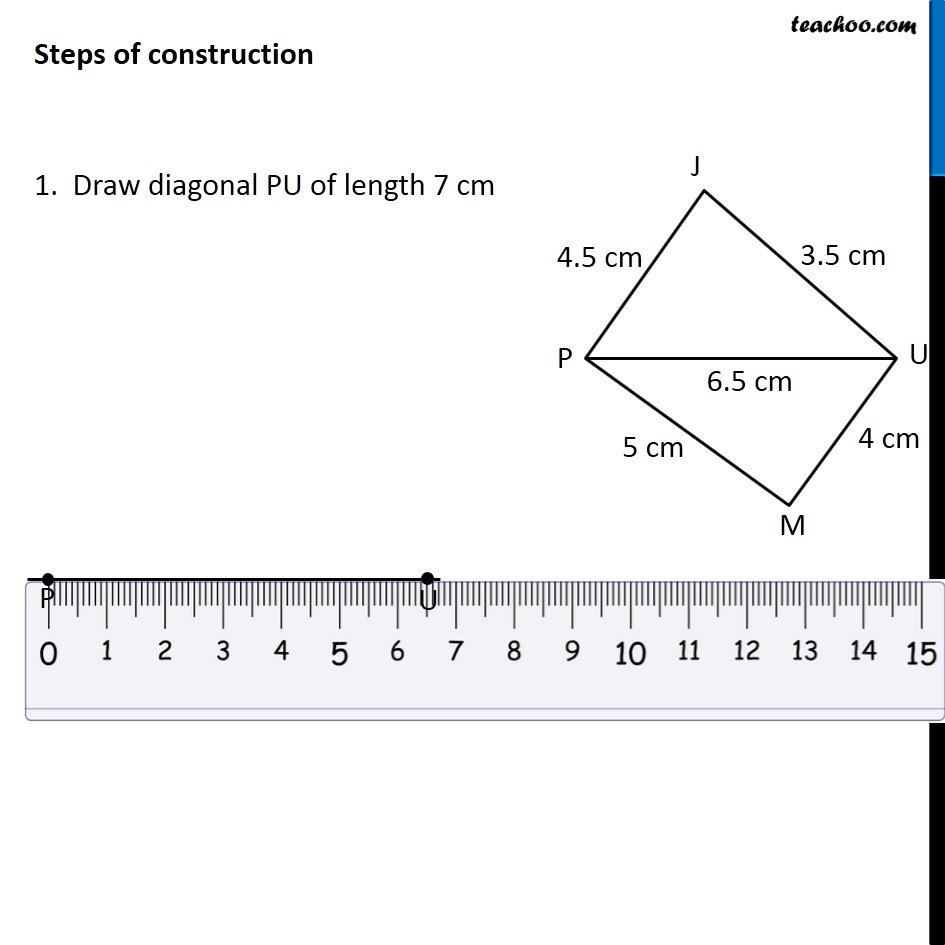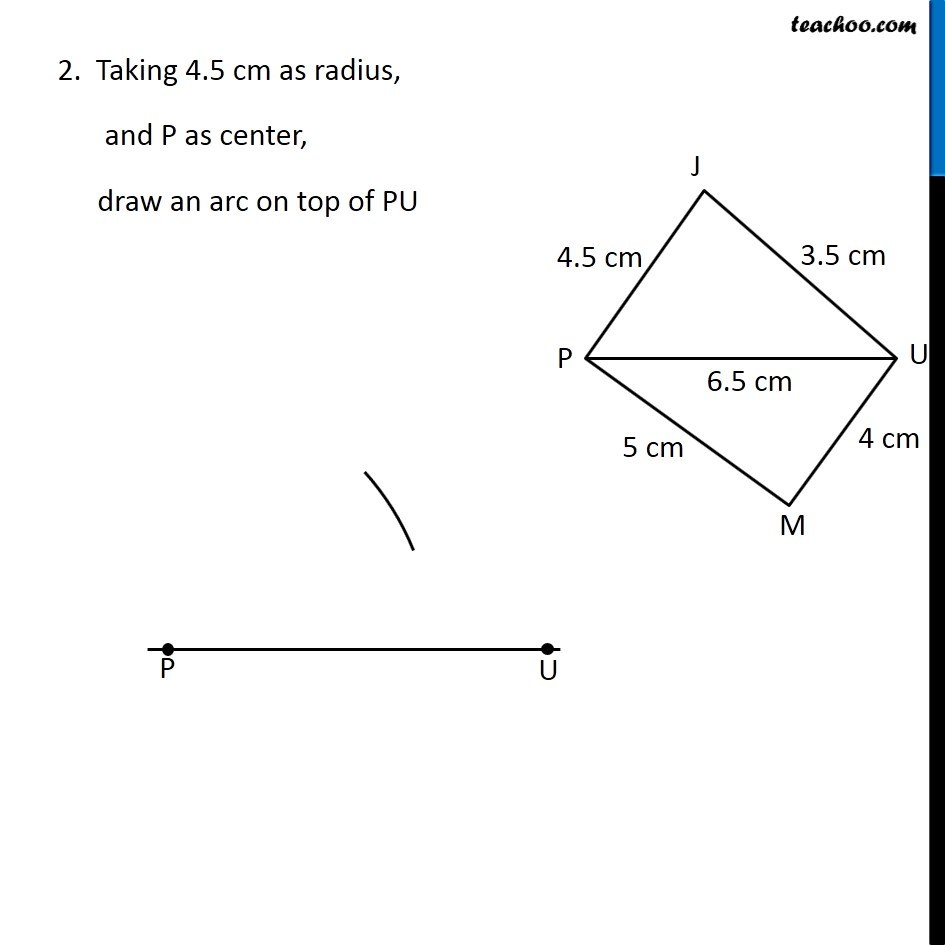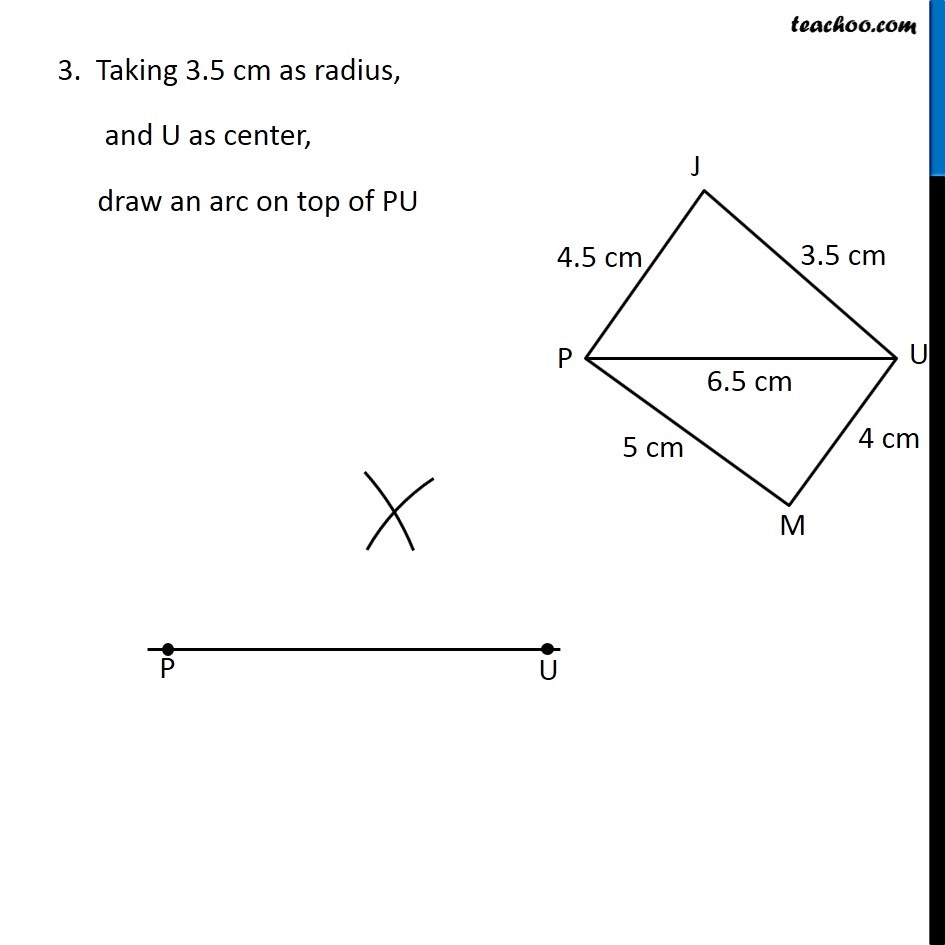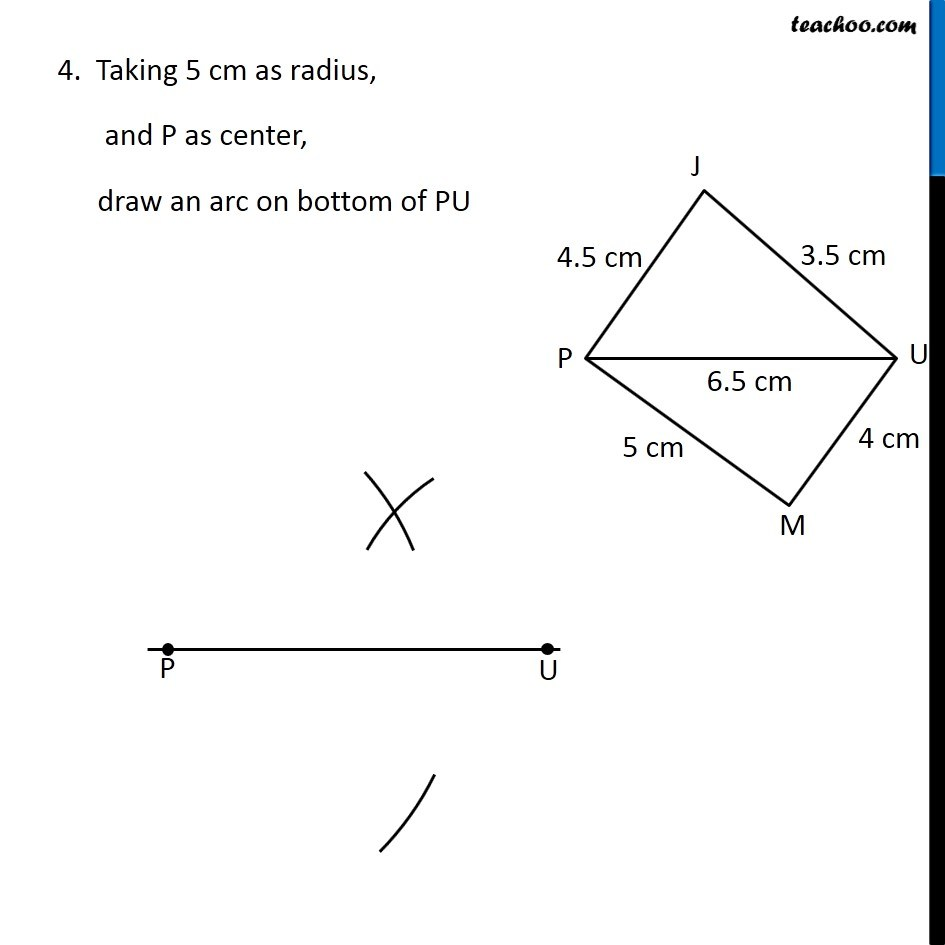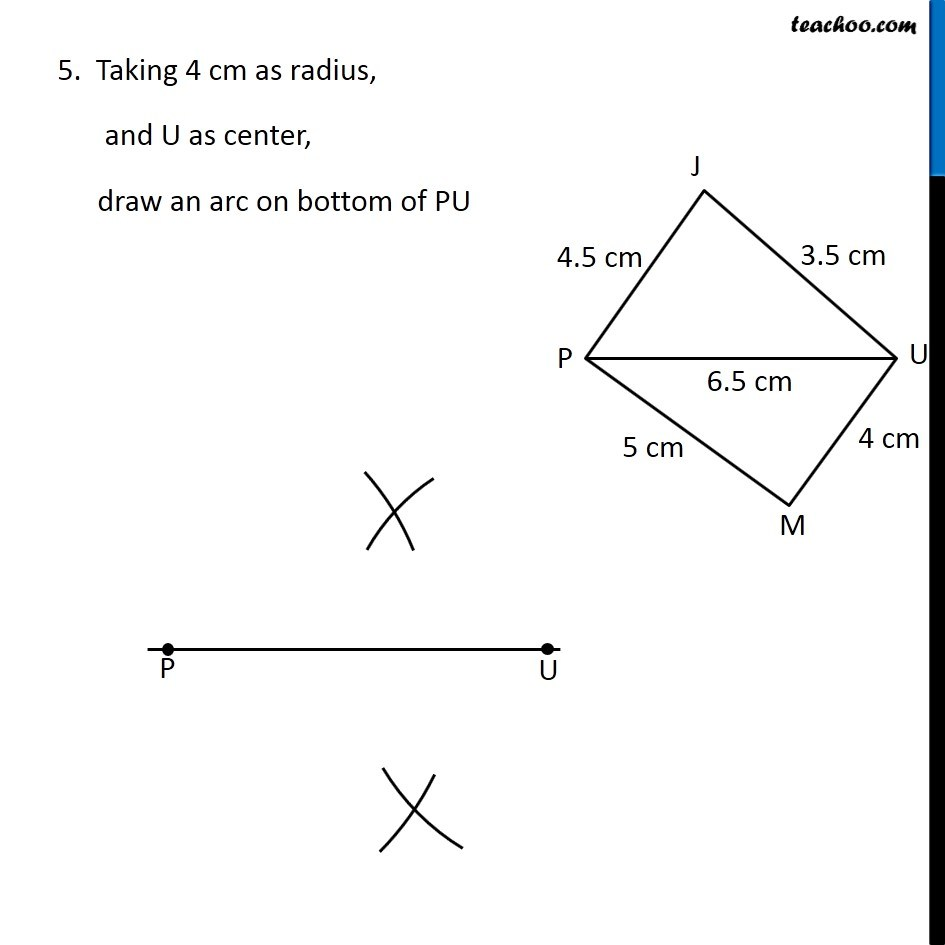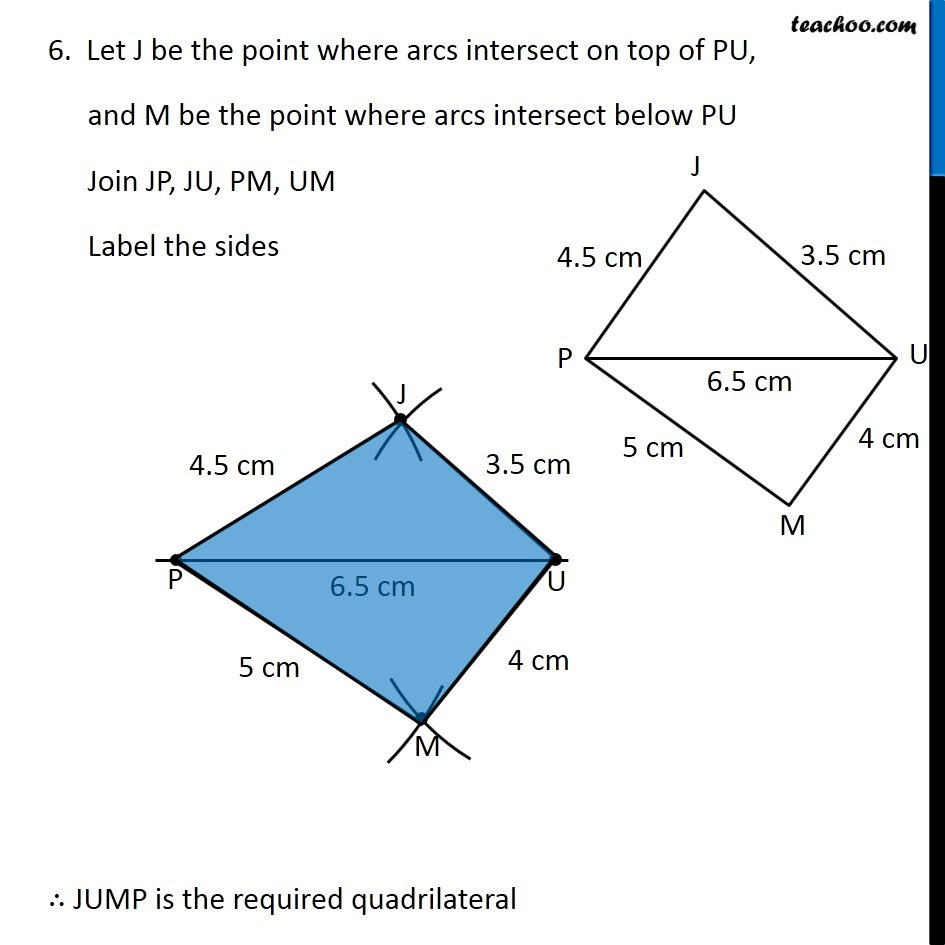1. Chapter 4 Class 8 Practical Geometry
2. Concept wise
3. Constructing Quadrilateral - 4 sides and diagonal given

Transcript

Ex 4.1, 1 (ii) Construct the following quadrilaterals. Quadrilateral JUMP JU = 3.5 cm UM = 4 cm MP = 5 cm PJ = 4.5 cm PU = 6.5 cm First we draw a rough sketch Let’s construct it Steps of construction 1. Draw diagonal PU of length 7 cm Steps of construction 1. Draw diagonal PU of length 7 cm 3. Taking 3.5 cm as radius, and U as center, draw an arc on top of PU 4. Taking 5 cm as radius, and P as center, draw an arc on bottom of PU 5. Taking 4 cm as radius, and U as center, draw an arc on bottom of PU 6. Let J be the point where arcs intersect on top of PU, and M be the point where arcs intersect below PU Join JP, JU, PM, UM Label the sides ∴ JUMP is the required quadrilateral

Constructing Quadrilateral - 4 sides and diagonal given